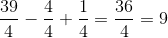Scan QR code or get instant email to install app

Question:

# 1212If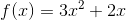and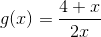, what is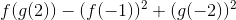?

A 9
explanation

1212Evaluate each of the functions with its respective input value and then perform the indicated operations to find the final value. First evaluate g(2) then use this as the input value of f(x):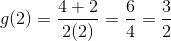. Substitute this value into f(x):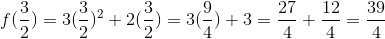Evaluate f(-1) and square the result: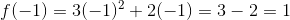, so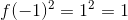Evaluate g(-2) and square the result: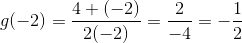, so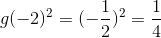And, substituting each of these values into the original combination of functions:, which becomes: